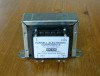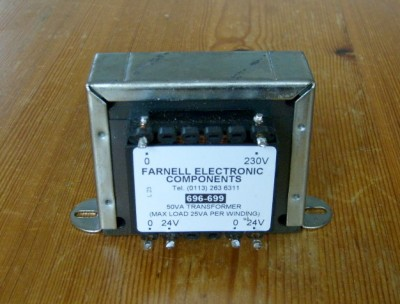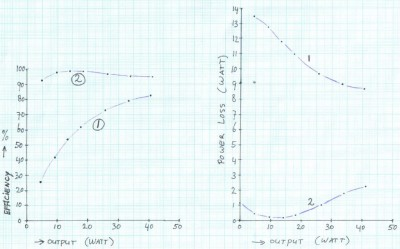OliNo

Renewable Energy

Saving energy with the toroidal transformerIn most electronic equipment, a low-cost EI-core transformer is used, which is much less efficient than a toroidal transformer. This article shows that, quite some energy can be saved by using a more efficient transformer.

In electronic equipment, a transformer is used to transform the mains voltage to another (most times lower) voltage. The transformer also provides a safe separation between mains voltage and the electronic circuit.

In many equipment a transformer with EI-core can be found, mainly because of the low price of such transformers. The name EI-core refers to the shape of the transformer core, which is built up with E and I shaped metal plates. A disadvantage of the EI-core transformer is the energy loss occurring in it, by which quite some energy is lost in heat production in the transformer. When using a transformer with toroidal core, the energy losses will be much lower. With the following measurements, this is demonstrated.

I measured the energy losses of the following two transformers:EI-core transformer 2x 24V 50 VAToroidal transformer 2x 9V 50VA

The transformers were loaded with several load resistors, with loading power ranging from 5 to 40 Watt. The input power of the transformer is measured with a rebuilt energy meter PM230 of brand: Brennenstuhl. The difference between input power and output power is the energy loss which is converted into heat in the transformer.

If we divide output power by input power, we get the efficiency of the transformer.

Table 1: Losses in EI-core transformer 2x 24 V 50 VA
resistors
parallel
Output voltage
(Volt a.c.)
Input power
(Watt)
Output power
(Watt)
Efficiency Power loss
(Watt)
9.1 0.000 0.000 9.100
1x 560 Ω 51.0 18.1 4.645 0.257 13.455
2x 560 Ω 50.6 21.9 9.144 0.418 12.756
3x 560 Ω 50.2 25.3 13.500 0.534 11.800
4x 560 Ω 49.8 28.7 17.715 0.617 10.985
6x 560 Ω 49.1 35.5 25.830 0.728 9.670
8x 560 Ω 48.5 42.6 33.604 0.789 8.996
10x 560 Ω 47.7 49.3 40.630 0.824 8.670
Table 2: Losses in toroidal transformer 2x 9 V 50 VA
resistors
parallel
Output voltage
(Volt a.c.)
Input power
(Watt)
Output power
(Watt)
Efficiency Power loss
(Watt)
1.16 0.000 0.000 1.160
1x 82 Ω 20.1 5.33 4.927 0.924 0.403
2x 82 Ω 20.0 9.97 9.756 0.979 0.214
3x 82 Ω 19.7 14.38 14.198 0.987 0.182
4x 82 Ω 19.4 18.70 18.359 0.982 0.341
6x 82 Ω 19.1 27.70 26.693 0.964 1.007
8x 82 Ω 18.7 35.90 34.116 0.950 1.784
10x 82 Ω 18.4 43.50 41.288 0.949 2.212This graphs show the results of the measurements.

The left graph shows the efficiency of the transformer.
The right graph shows the power loss in the transformer.
Line 1 is for the EI-core transformer.
Line 2 is for the toroidal transformer.

We see the toroidal transformer gives much less losses then the EI-core transformer.

Encouraged by these results, I replaced in my modem and aerial amplifier the EI-core transformer with a toroidal transformer as well as some other energy saving measures. Through this I save tens of kWh per year.